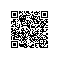# WPF自定义控件的三种方式

WPF控件可以通过数据模型（DataTemplate）、样式(Style)、控件模板（ControlTemplate)和触发器(Trigger)等机制减少创建新控件的需要。 但是，某些场景下，我们确实需要创建新的控件。此时，理解 WPF不同控件的创建方法就显得非常重要。 WPF 提供3个用于创建控件的方法，每个方法都提供不同的灵活度，下面分别进行介绍。

### 4 依赖属性

WPF 可以通过设置控件的属性来更改其外观和行为。其中的依赖属性可以让自定义控件执行以下操作：

• 在样式中设置该属性。
• 将该属性绑定到数据源。
• 使用动态资源作为该属性的值。
• 对该属性进行动画处理。

/// <summary>
/// Identifies the Value dependency property.
/// </summary>
public static readonly DependencyProperty ValueProperty =
DependencyProperty.Register(
"Value", typeof(decimal), typeof(NumericUpDown),
new CoerceValueCallback(CoerceValue)));

/// <summary>
/// Gets or sets the value assigned to the control.
/// </summary>
public decimal Value
{
get { return (decimal)GetValue(ValueProperty); }
set { SetValue(ValueProperty, value); }
}

private static object CoerceValue(DependencyObject element, object value)
{
decimal newValue = (decimal)value;
NumericUpDown control = (NumericUpDown)element;

newValue = Math.Max(MinValue, Math.Min(MaxValue, newValue));

return newValue;
}

private static void OnValueChanged(DependencyObject obj, DependencyPropertyChangedEventArgs args)
{
NumericUpDown control = (NumericUpDown)obj;

RoutedPropertyChangedEventArgs<decimal> e = new RoutedPropertyChangedEventArgs<decimal>(
(decimal)args.OldValue, (decimal)args.NewValue, ValueChangedEvent);
control.OnValueChanged(e);
}

• 属性的名称Value
• 属性的类型decimal
• 拥有属性的类型NumericUpDown使用钉钉扫一扫加入圈子
+ 订阅